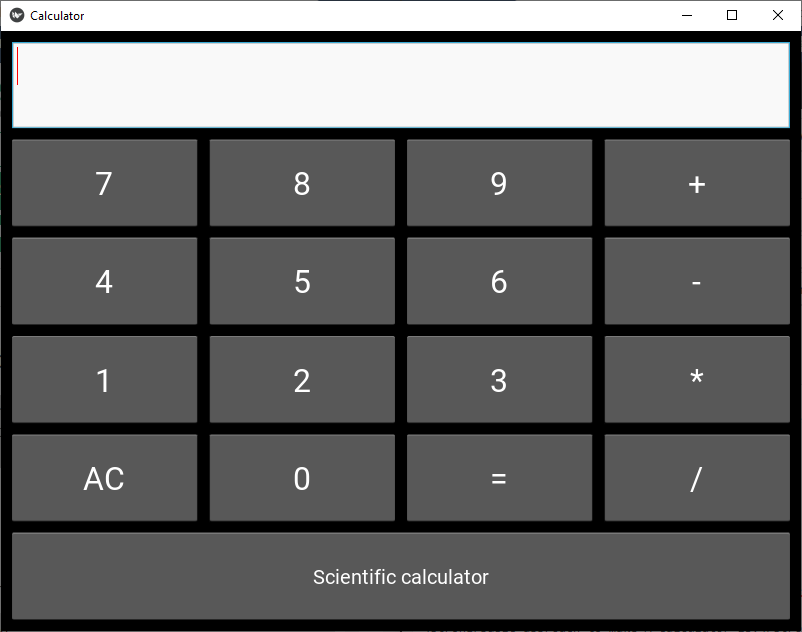# How to make calculator using kivy | Python

Kivy is a platform-independent GUI tool in Python. As it can be run on Android, IOS, linux and Windows etc. It is basically used to develop the Android application, but it does not mean that it can not be used on Desktops applications.

In this article, we will learn how to make a simple calculator using Kivy.

Prerequisites:

1) Basic knowledge of Mathematics
2) Python
3) Kivy
4) Widgets and code understanding of kivy

```Basic approach to make A calculator:

1) import kivy
2) import kivyApp
3) import Gridlayout
4) import config(to configure/adjust the window size)
5) Set minimum version(optional)
6) Create Layout class :
define Calculator function in it
: In this i am using try-catch because if any arithmetic
exception occur it will through the error

7) create App class
8) create .kv file (name same as the app class):
1) create buttons
2) Add the style to the buttons
3) Add fuctionalties of the button
9) return Layout/widget/Class(according to requirement)
10) Run an instance of the class```

Implementation of the Approach:

main.py

 `# Program to create a calculator  ` `   `  `# Program to Show how to create a switch  ` `# import kivy module     ` `import` `kivy   ` `      `  `# base Class of your App inherits from the App class.     ` `# app:always refers to the instance of your application    ` `from` `kivy.app ``import` `App  ` `    `  `# this restrict the kivy version i.e   ` `# below this kivy version you cannot   ` `# use the app or software   ` `kivy.require(``'1.9.0'``)  ` ` `  `# for making multiple bttons to arranging ` `# them we are using this ` `from` `kivy.uix.gridlayout ``import` `GridLayout ` ` `  `# for the size of window ` `from` `kivy.config ``import` `Config ` ` `  `# Setting size to resizable ` `Config.``set``(``'graphics'``, ``'resizable'``, ``1``) ` `## Config.set('graphics', 'width', '400') ` `## Config.set('graphics', 'height', '400') ` ` `  ` `  `# Creating Layout class ` `class` `CalcGridLayout(GridLayout): ` `  `  `    ``# Function called when equals is pressed ` `    ``def` `calculate(``self``, calculation): ` `        ``if` `calculation: ` `            ``try``: ` `                ``# Solve formula and display it in entry ` `                ``# which is pointed at by display ` `                ``self``.display.text ``=` `str``(``eval``(calculation)) ` `            ``except` `Exception: ` `                ``self``.display.text ``=` `"Error"` `  `  ` ``# Creating App class ` `class` `CalculatorApp(App): ` `  `  `    ``def` `build(``self``): ` `        ``return` `CalcGridLayout() ` `  `  `# creating object and running it  ` `calcApp ``=` `CalculatorApp() ` `calcApp.run() ` ` `

calculator.kv

 `# Custom button ` `: ` `    ``font_size: ``32` `  `  `# Define id so I can refer to the CalcGridLayout ` `# class functions ` `# Display points to the entry widget ` `: ` `    ``id``: calculator ` `    ``display: entry ` `    ``rows: ``6` `    ``padding: ``10` `    ``spacing: ``10` ` `  `     `  `  `  `    ``# Where input is displayed ` `    ``BoxLayout: ` `        ``TextInput: ` `            ``id``: entry ` `            ``font_size: ``32` `            ``multiline: ``False` `  `  `    ``# When buttons are pressed update the entry ` `    ``BoxLayout: ` `        ``spacing: ``10` `        ``CustButton: ` `            ``text: ``"7"` `            ``on_press: entry.text ``+``=` `self``.text ` `        ``CustButton: ` `            ``text: ``"8"` `            ``on_press: entry.text ``+``=` `self``.text ` `        ``CustButton: ` `            ``text: ``"9"` `            ``on_press: entry.text ``+``=` `self``.text ` `        ``CustButton: ` `            ``text: ``"+"` `            ``on_press: entry.text ``+``=` `self``.text ` `  `  `    ``BoxLayout: ` `        ``spacing: ``10` `        ``CustButton: ` `            ``text: ``"4"` `            ``on_press: entry.text ``+``=` `self``.text ` `        ``CustButton: ` `            ``text: ``"5"` `            ``on_press: entry.text ``+``=` `self``.text ` `        ``CustButton: ` `            ``text: ``"6"` `            ``on_press: entry.text ``+``=` `self``.text ` `        ``CustButton: ` `            ``text: ``"-"` `            ``on_press: entry.text ``+``=` `self``.text ` `  `  `    ``BoxLayout: ` `        ``spacing: ``10` `        ``CustButton: ` `            ``text: ``"1"` `            ``on_press: entry.text ``+``=` `self``.text ` `        ``CustButton: ` `            ``text: ``"2"` `            ``on_press: entry.text ``+``=` `self``.text ` `        ``CustButton: ` `            ``text: ``"3"` `            ``on_press: entry.text ``+``=` `self``.text ` `        ``CustButton: ` `            ``text: ``"*"` `            ``on_press: entry.text ``+``=` `self``.text ` `  `  `    ``# When equals is pressed pass text in the entry ` `    ``# to the calculate function ` `    ``BoxLayout: ` `        ``spacing: ``10` `        ``CustButton: ` `            ``text: ``"AC"` `            ``on_press: entry.text ``=` `"" ` `        ``CustButton: ` `            ``text: ``"0"` `            ``on_press: entry.text ``+``=` `self``.text ` `        ``CustButton: ` `            ``text: ``"="` `            ``on_press: calculator.calculate(entry.text) ` `        ``CustButton: ` `            ``text: ``"/"` `            ``on_press: entry.text ``+``=` `self``.text ` `    ``BoxLayout: ` `        ``CustButton: ` `            ``font_size: ``20` `            ``text: ``"Scientific calculator"` `            ``on_press: entry.text ``=` `""         `

Output:Attention geek! Strengthen your foundations with the Python Programming Foundation Course and learn the basics.

To begin with, your interview preparations Enhance your Data Structures concepts with the Python DS Course.

My Personal Notes arrow_drop_upCompetative Programmer

If you like GeeksforGeeks and would like to contribute, you can also write an article using contribute.geeksforgeeks.org or mail your article to contribute@geeksforgeeks.org. See your article appearing on the GeeksforGeeks main page and help other Geeks.

Please Improve this article if you find anything incorrect by clicking on the "Improve Article" button below.

Article Tags :

Be the First to upvote.

Please write to us at contribute@geeksforgeeks.org to report any issue with the above content.